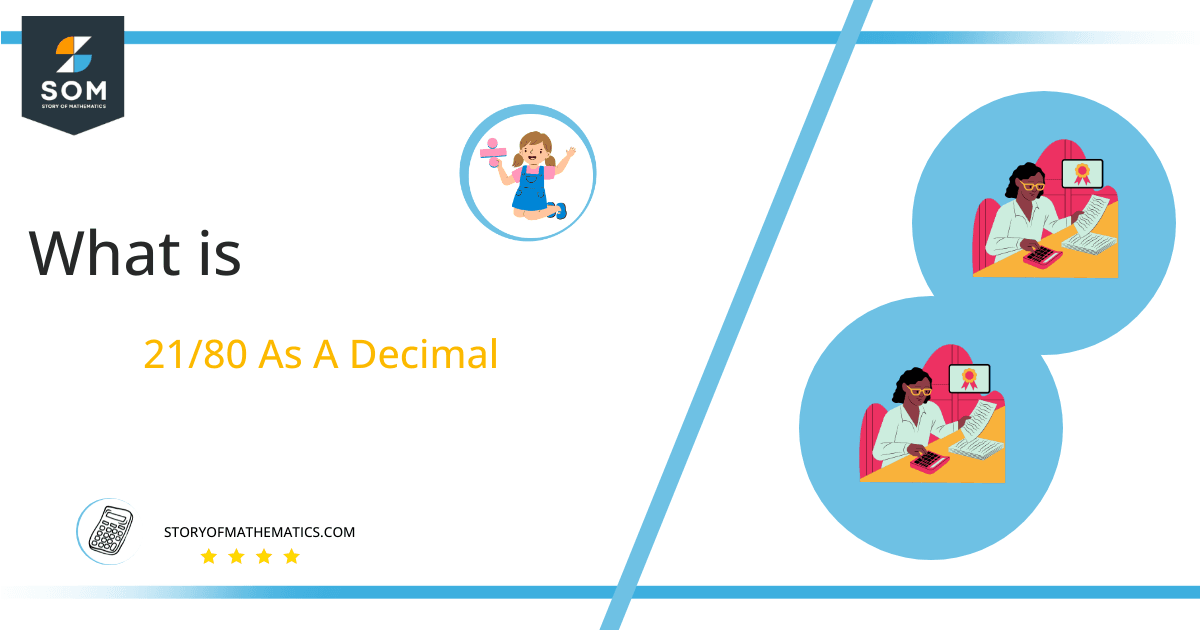# What Is 21/80 as a Decimal + Solution With Free Steps

The fraction 21/80 as a decimal is equal to 0.262.

Rational numbers can be expressed in the form of fractions. The fraction 21/80 represents a rational number in which 21 is the numerator and 80 is the denominator. As the numerator is less than the denominator, it is a proper fraction.

Here, we are more interested in the division types that result in a Decimal value, as this can be expressed as a Fraction. We see fractions as a way of showing two numbers having the operation of Division between them that result in a value that lies between two Integers.Now, we introduce the method used to solve said fraction to decimal conversion, called Long Division, which we will discuss in detail moving forward. So, let’s go through the Solution of fraction 21/80.

## Solution

First, we convert the fraction components, i.e., the numerator and the denominator, and transform them into the division constituents, i.e., the Dividend and the Divisor, respectively.

This can be done as follows:

Dividend = 21

Divisor = 80

Now, we introduce the most important quantity in our division process: the Quotient. The value represents the Solution to our division and can be expressed as having the following relationship with the Division constituents:

Quotient = Dividend $\div$ Divisor = 21 $\div$ 80

This is when we go through the Long Division solution to our problem. The following figure shows the solution for fraction 21/80.Figure 1

## 21/80 Long Division Method

We start solving a problem using the Long Division Method by first taking apart the division’s components and comparing them. As we have 21 and 80, we can see how 21 is Smaller than 80, and to solve this division, we require that 21 be Bigger than 80.

This is done by multiplying the dividend by 10 and checking whether it is bigger than the divisor or not. If so, we calculate the Multiple of the divisor closest to the dividend and subtract it from the Dividend. This produces the Remainder, which we then use as the dividend later.

Now, we begin solving for our dividend 21, which after getting multiplied by 10 becomes 210.

We take this 210 and divide it by 80; this can be done as follows:

210 $\div$ 80 $\approx$ 2

Where:

80 x 2 = 160

This will lead to the generation of a Remainder equal to 210 – 160 = 50. Now this means we have to repeat the process by Converting the 50 into 500 and solving for that:

500 $\div$ 80 $\approx$ 6

Where:

80 x 6 = 480

This, therefore, produces another Remainder which is equal to 500 – 480 = 20. Now this means we have to repeat the process by Converting the 20 into 200 and solving for that:

200 $\div$ 80 $\approx$ 2

Where:

80 x 2 = 160

Finally, we have a Quotient generated after combining the three pieces of it as 0.262, with a Remainder equal to 40.Images/mathematical drawings are created with GeoGebra.